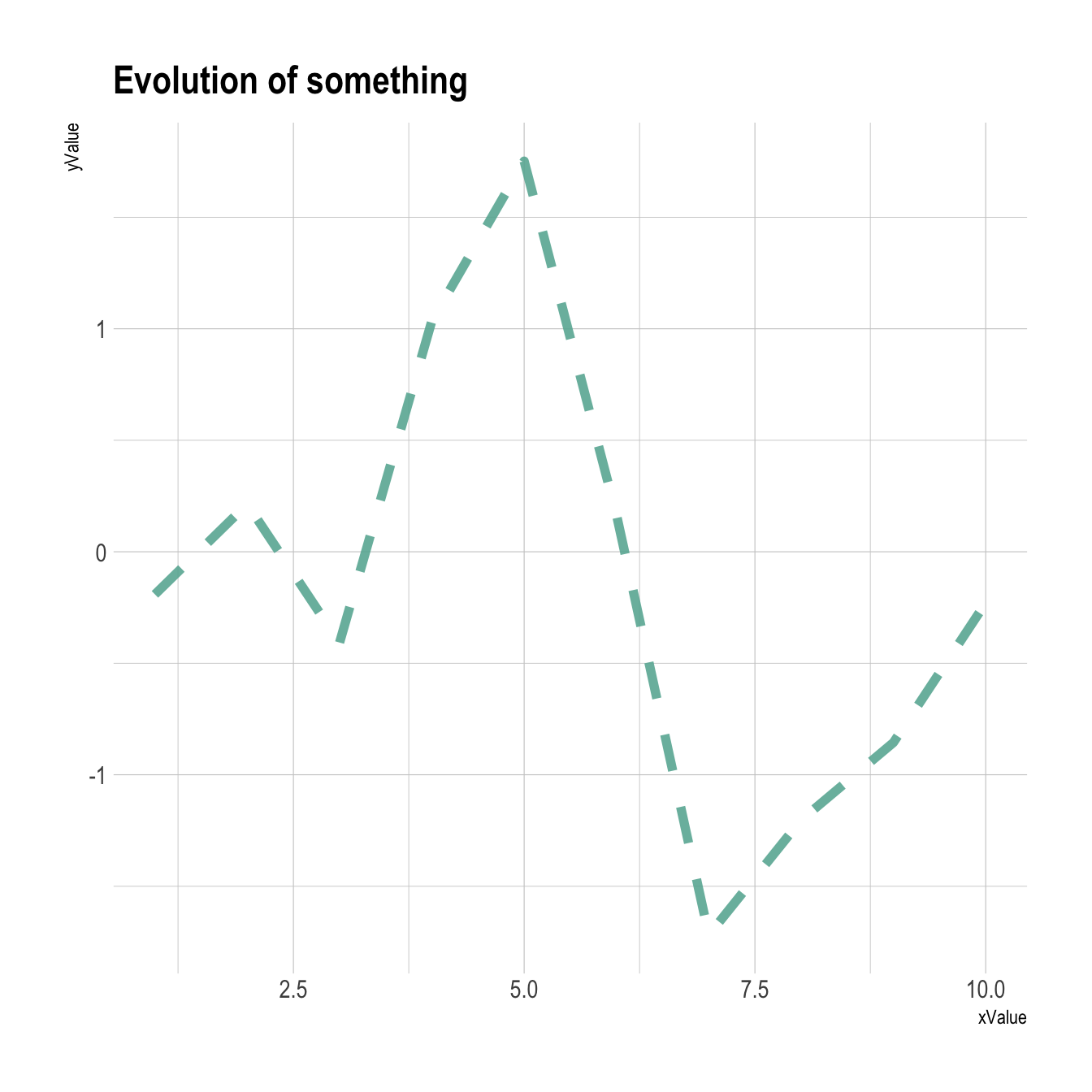# Line chart with R and ggplot2

This post is a step by step introduction to line chart with R and ggplot2. It provides several reproducible examples with explanation and R code.

# Basic line chart with `ggplot2` and `geom_line()`

A line chart or line graph displays the evolution of one or several numeric variables. Data points are usually connected by straight line segments. You read an extensive definition here.

The input data frame requires at least 2 columns:

• An ordered numeric variable for the X axis
• Another numeric variable for the Y axis

Once the data is read by ggplot2 and those 2 variables are specified in the `x` and `y` arguments of the `aes()`, just call the `geom_line()` function.``````# Libraries
library(ggplot2)

# create data
xValue <- 1:10
yValue <- cumsum(rnorm(10))
data <- data.frame(xValue,yValue)

# Plot
ggplot(data, aes(x=xValue, y=yValue)) +
geom_line()``````

# Customize the line chart

Several options are available to customize the line chart appearance:

• Add a title with `ggtitle()`.
• Change line style with arguments like `shape`, `size`, `color` and more.
• Custom the general theme with the `theme_ipsum()` function of the `hrbrthemes` package.

More generally, visit the [ggplot2 section] for more ggplot2 related stuff.``````# Libraries
library(ggplot2)
library(hrbrthemes)

# create data
xValue <- 1:10
yValue <- cumsum(rnorm(10))
data <- data.frame(xValue,yValue)

# Plot
ggplot(data, aes(x=xValue, y=yValue)) +
geom_line( color="#69b3a2", size=2, alpha=0.9, linetype=2) +
theme_ipsum() +
ggtitle("Evolution of something")``````

Related chart types

## Contact

This document is a work by Yan Holtz. Any feedback is highly encouraged. You can fill an issue on Github, drop me a message on Twitter, or send an email pasting yan.holtz.data with gmail.com.